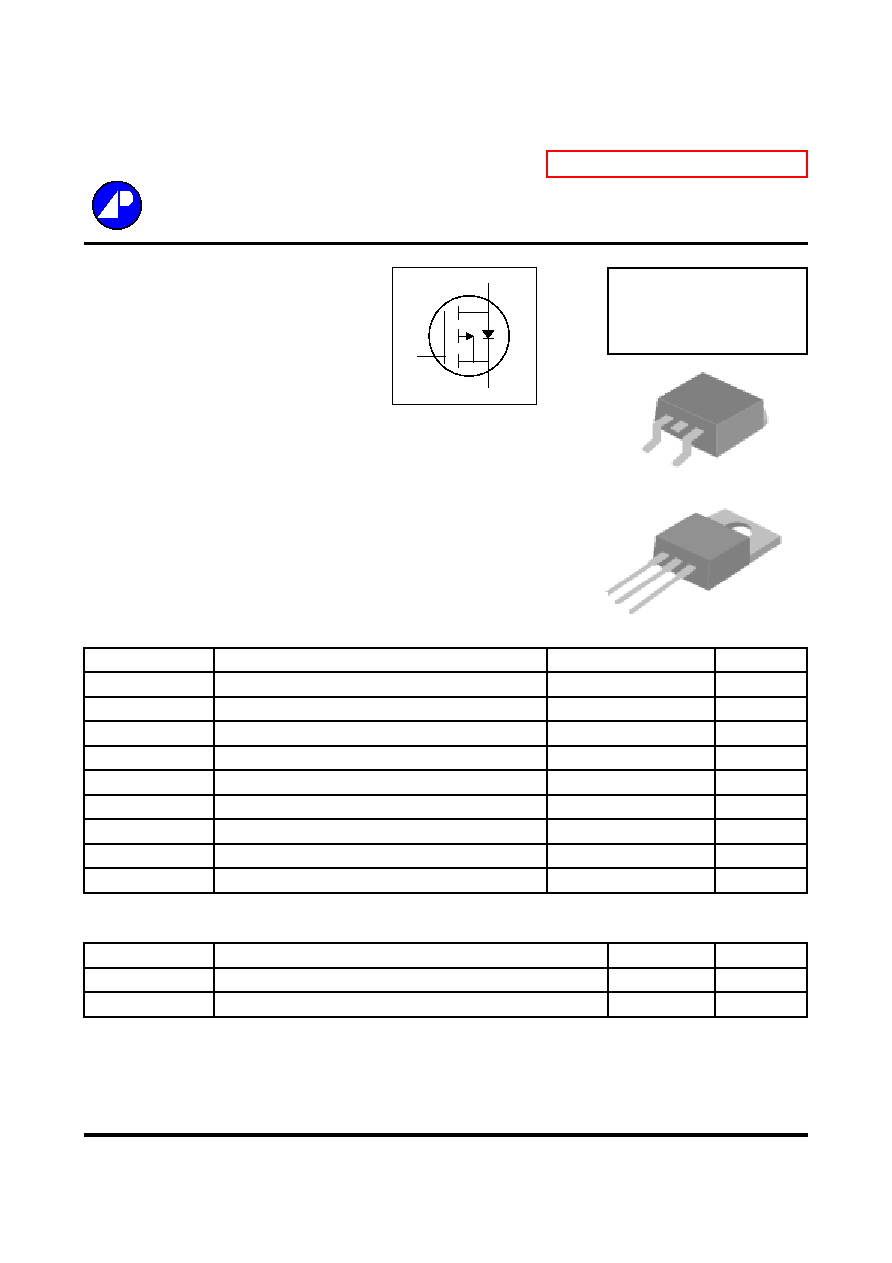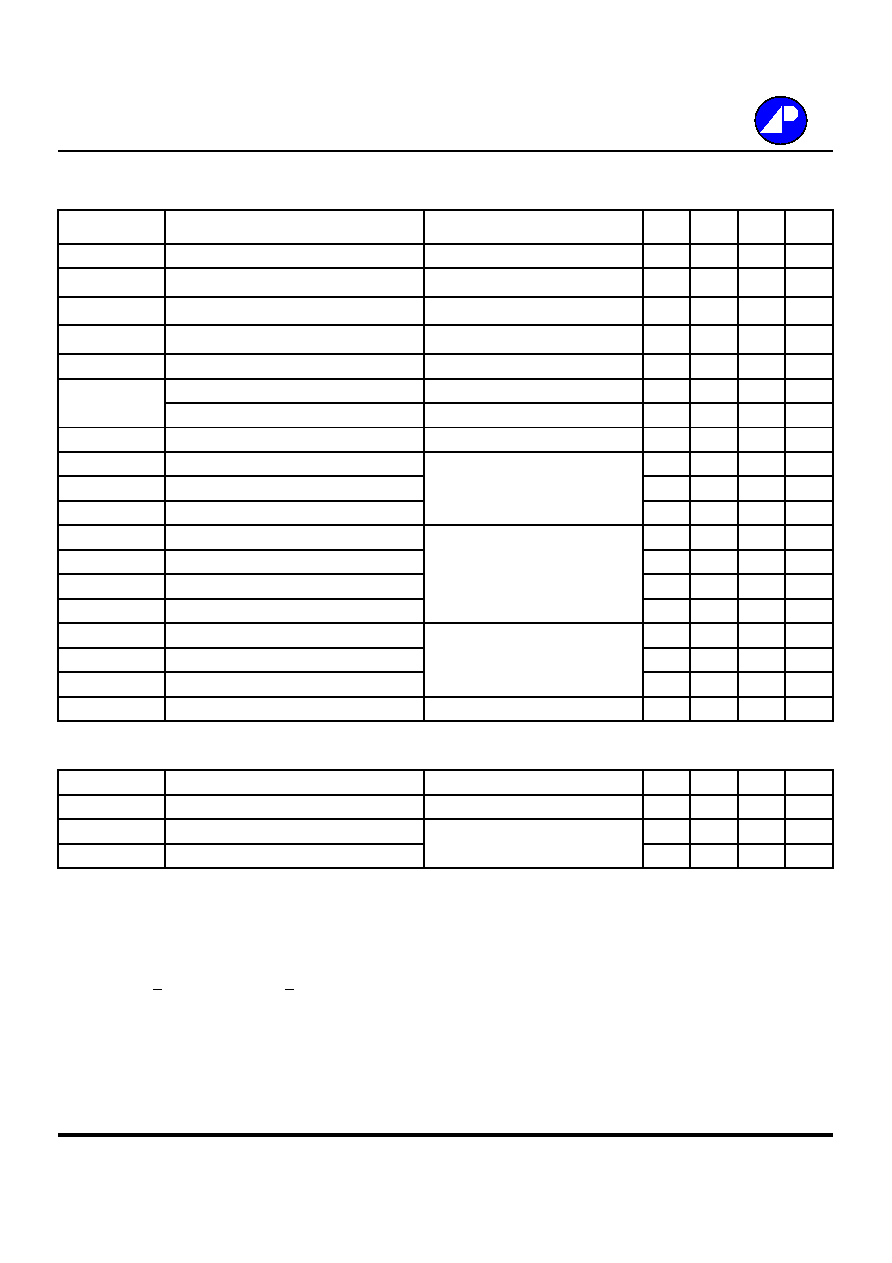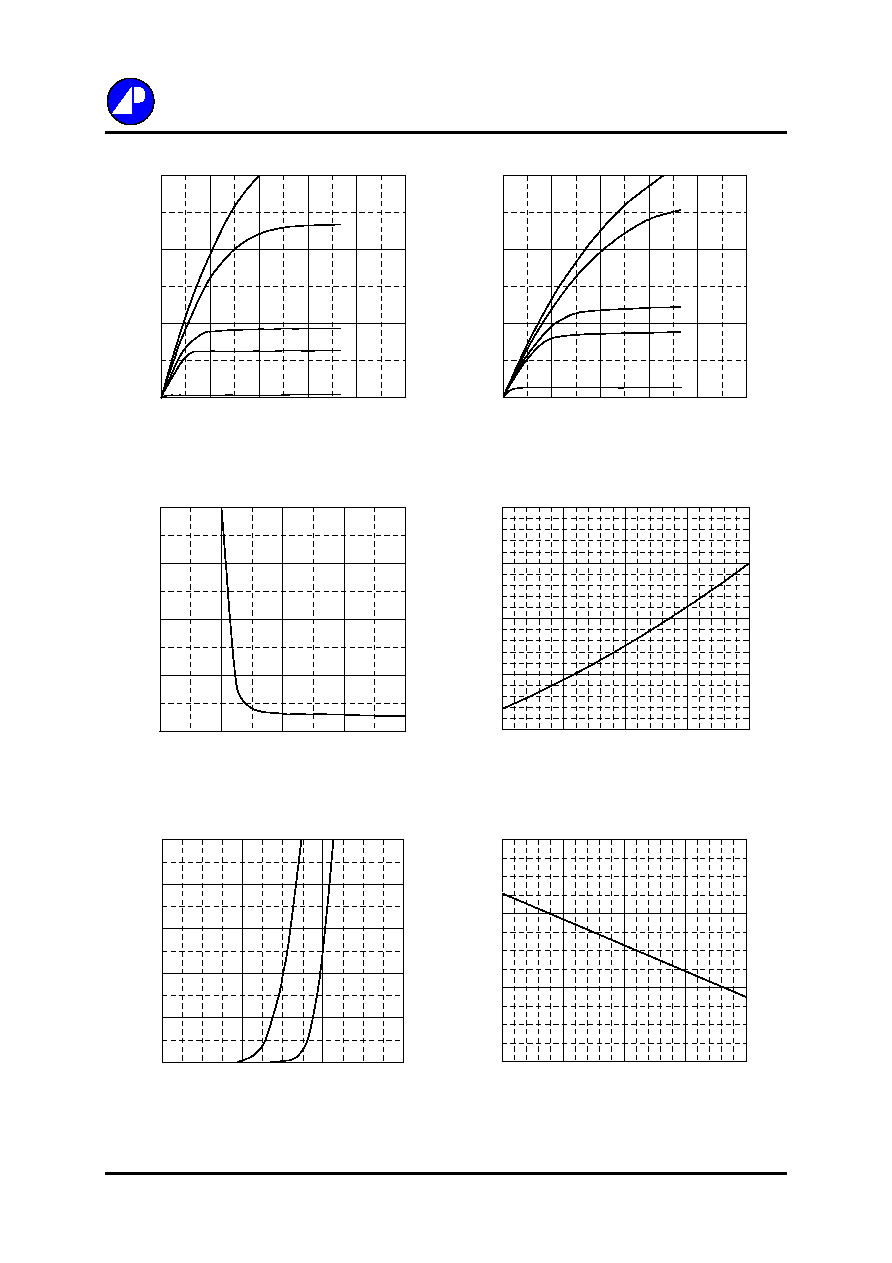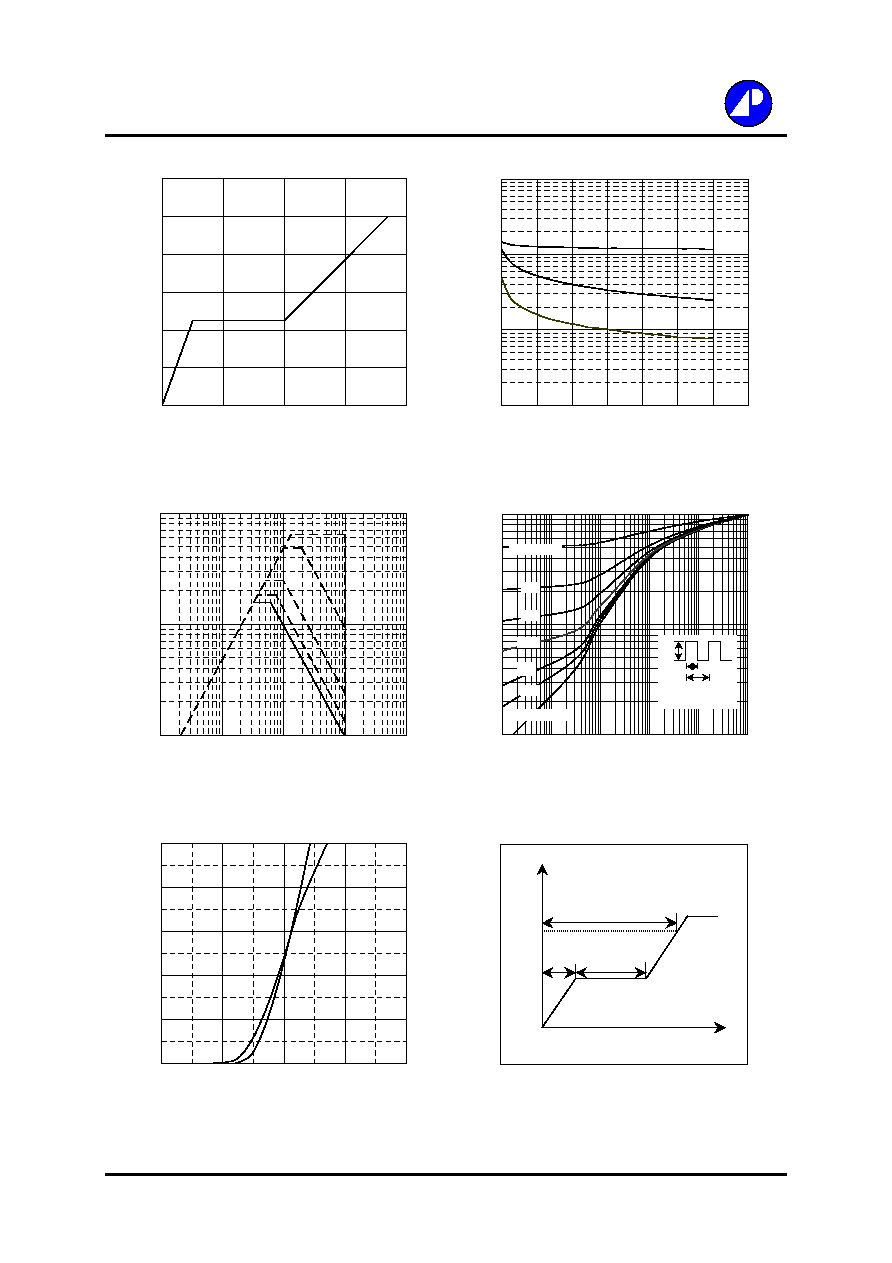Search datasheet (1.687.043 components) Search fieldPart namePart descriptionAbout site Manufacturers list ChipFindIC search engine AllXrefCross-reference database### Datasheet: AP15P10GP (Advanced Power Electronics Corp.)

P-channel Enhancement Mode Power Mosfet
Download:PDFZIPP-CHANNEL ENHANCEMENT MODE
Electronics Corp.
POWER MOSFET
Lower On-resistance
BV
DSS
-100V
Simple Drive Requirement
R
DS(ON)
210m
Fast Switching Characteristic
I
D
-16A
RoHS Compliant
Description
Absolute Maximum Ratings
Symbol
Units
V
DS
V
V
GS
V
I
D
@T
C
=25
A
I
D
@T
C
=100
A
I
DM
A
P
D
@T
C
=25
W
W/
T
STG
T
J
Symbol
Value
Units
Rthj-c
Thermal Resistance Junction-case
Max.
1.3
/W
Rthj-a
Thermal Resistance Junction-ambient
Max.
62
/W
Data and specifications subject to change without notice
200728051-1/4
AP15P10GS/P
Rating
-100
�20
-16
0.77
Pb Free Plating Product
Continuous Drain Current, V
GS
@ 10V
-9.8
Pulsed Drain Current
1
64
Thermal Data
Parameter
Total Power Dissipation
Operating Junction Temperature Range
Storage Temperature Range
-55 to 150
Linear Derating Factor
96
-55 to 150
Parameter
Drain-Source Voltage
Gate-Source Voltage
Continuous Drain Current, V
GS
@ 10V
G
D
S
The Advanced Power MOSFETs from APEC provide the
designer with the best combination of fast switching,
ruggedized device design, low on-resistance and cost-effectiveness.
The TO-263 package is universally preferred for all commercial-
industrial surface mount applications and suited for low voltage
applications such as DC/DC converters. The through-hole version
(AP15P10GP) are available for low-profile applications.
G D
S
TO-263(S)
G
D
S
TO-220(P)AP15P10GS/P
Electrical Characteristics@T
j
=25
o
C(unless otherwise specified)
Symbol
Parameter
Test Conditions
Min.
Typ. Max. Units
BV
DSS
Drain-Source Breakdown Voltage
V
GS
=0V, I
D
=-1mA
-100
-
-
V
B
V
DSS
/T
j
Breakdown Voltage Temperature Coefficient
Reference to 25
, I
D
=-1mA
-
-0.1
-
V/
R
DS(ON)
Static Drain-Source On-Resistance
2
V
GS
=-10V, I
D
=-9A
-
-
210
m
V
GS(th)
Gate Threshold Voltage
V
DS
=V
GS
, I
D
=-250uA
-1
-
-3
V
g
fs
Forward Transconductance
V
DS
=-10V, I
D
=-9A
-
8
-
S
I
DSS
Drain-Source Leakage Current (T
j
=25
o
C)
V
DS
=-100V, V
GS
=0V
-
-
-25
uA
Drain-Source Leakage Current (T
j
=150
o
C)
V
DS
=-80V, V
GS
=0V
-
-
-100
uA
I
GSS
Gate-Source Leakage
V
GS
= �20V
-
-
�100
nA
Q
g
Total Gate Charge
2
I
D
=-9A
-
37
60
nC
Q
gs
Gate-Source Charge
V
DS
=-80V
-
5
-
nC
Q
gd
Gate-Drain ("Miller") Charge
V
GS
=-10V
-
15
-
nC
t
d(on)
Turn-on Delay Time
2
V
DS
=-50V
-
11
-
ns
t
r
Rise Time
I
D
=-9A
-
25
-
ns
t
d(off)
Turn-off Delay Time
R
G
=10
,
V
GS
=-10V
-
56
-
ns
t
f
Fall Time
R
D
=5.6
-
36
-
ns
C
iss
Input Capacitance
V
GS
=0V
-
1180 1900
pF
C
oss
Output Capacitance
V
DS
=-25V
-
250
-
pF
C
Reverse Transfer Capacitance
f=1.0MHz
-
75
-
pF
R
g
Gate Resistance
f=1.0MHz
-
3.6
5
Source-Drain Diode
Symbol
Parameter
Test Conditions
Min.
Typ. Max. Units
V
SD
Forward On Voltage
2
I
S
=-9A, V
GS
=0V
-
-
-1.3
V
t
rr
Reverse Recovery Time
2
I
S
=-9A, V
GS
=0V,
-
95
-
ns
Q
rr
Reverse Recovery Charge
dI/dt=-100A/�s
-
410
-
nC
Notes:
1.Pulse width limited by safe operating area.
2.Pulse width <300us , duty cycle <2%.
2/4AP15P10GS/P
Fig 1. Typical Output Characteristics
Fig 2. Typical Output Characteristics
Fig 3. On-Resistance v.s. Gate Voltage
Fig 4. Normalized On-Resistance
v.s. Junction Temperature
Fig 5. Forward Characteristic of
Fig 6. Gate Threshold Voltage v.s.
Reverse Diode
Junction Temperature
3/4
0
10
20
30
0
5
10
15
20
25
-V
DS
, Drain-to-Source Voltage (V)
-I
D
, Drain Current (A)
T
C
=25
o
C
-10V
-7.0V
-5.0V
-4.5V
V
G
= - 3 .0V
0
10
20
30
0
5
10
15
20
25
-V
DS
, Drain-to-Source Voltage (V)
-I
D
, Drain Current (A)
T
C
= 1 5 0
o
C
-10V
-7.0V
-5.0V
-4.5V
V
G
= - 3 .0V
150
250
350
450
550
2
4
6
8
10
-V
GS
, Gate-to-Source Voltage (V)
R
DS(
ON)
(m

)
I
D
= -9 A
T
C
=25
0.4
0.9
1.4
1.9
2.4
-50
0
50
100
150
T
j
, Junction Temperature (
o
C)
Norm
alize
d
R
DS(
ON)
I
D
= -9 A
V
G
= - 10V
0.3
0.7
1.1
1.5
-50
0
50
100
150
T
j
, Junction Temperature (
o
C)
Norm
alize
d
-
V
GS(
t
h)
(V)
0
2
4
6
8
10
0
0.4
0.8
1.2
-V
SD
, Source-to-Drain Voltage (V)
-I
S
(A)
T
j
=25
o
C
T
j
=150
o
CFig 7. Gate Charge Characteristics
Fig 8. Typical Capacitance Characteristics
Fig 9. Maximum Safe Operating Area
Fig 10. Effective Transient Thermal Impedance
Fig 11. Transfer Characteristics
Fig 12. Gate Charge Waveform
4/4
AP15P10GS/P
Q
V
G
-10V
Q
GS
Q
GD
Q
G
Charge
1
10
100
0.1
1
10
100
1000
-V
DS
, Drain-to-Source Voltage (V)
-I
D
(A
)
10ms
100ms
1s
DC
T
C
=25
o
C
Single Pulse
0
2
4
6
8
10
12
0
10
20
30
40
Q
G
, Total Gate Charge (nC)
-V
GS
,
G
a
te
to S
o
u
r
c
e
Voltage
(
V
)
I
D
= -9A
V
DS
= -80V
10
100
1000
10000
1
5
9
13
17
21
25
29
-V
DS
, Drain-to-Source Voltage (V)
C (
p
F)
f=1.0MHz
C
iss
C
oss
C
0
2
4
6
8
10
0
2
4
6
8
-V
GS
, Gate-to-Source Voltage (V)
-I
D
, Dr
a
i
n C
u
r
r
e
nt
(A
)
T
j
=150
o
C
T
j
=25
o
C
V
DS
=-5V
0.01
0.1
1
0.00001
0.0001
0.001
0.01
0.1
1
t , Pulse Width (s)
N
o
rmalize
d
T
h
e
r
mal Re
spon
se
(
R
th
jc
)
P
DM
Duty factor = t/T
Peak T
j
= P
DM
x R
thjc
+ T
C
t
T
0.02
0.01
0.05
0.1
0.2
Duty factor=0.5
Single Pulse© 2020 • ICSheet• Contact form• Main page